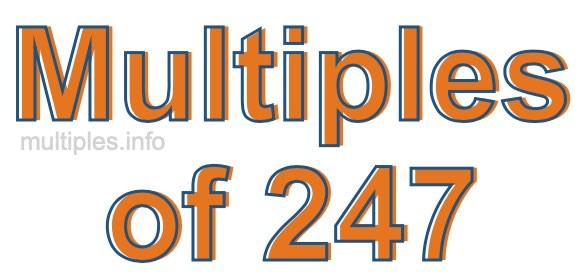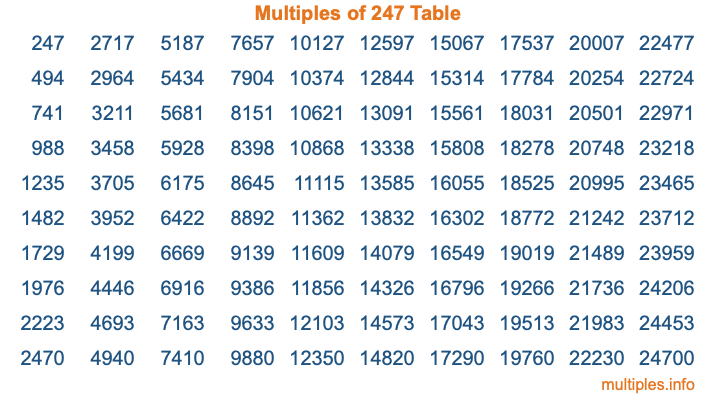Multiples of 247Welcome to the Multiples of 247 page. Here we will first teach you everything you will ever need to know about the multiples of 247, and then give you a study guide summary of everything we taught you to make sure you remember it all. Use this page to look up facts and learn information about the multiples of 247. This page will make you a multiples of two hundred forty-seven expert!

Definition of Multiples of 247
Multiples of 247 are all the numbers that when divided by 247 equal an integer. Each of the multiples of 247 are called a multiple. A multiple of 247 is created by multiplying 247 by an integer.

Therefore, to create a list of multiples of 247, you start with 1 multiplied by 247, then 2 multiplied by 247, then 3 multiplied by 247, and so on for as long as you want. Thus, the list of the first five multiples of 247 is 247, 494, 741, 988, and 1235. To see a larger list of multiples of 247, see the printable image of Multiples of 247 further down on this page. We also have a category where you can choose any nth multiple of 247.

Multiples of 247 Checker
The Multiples of 247 Checker below checks to see if any number of your choice is a multiple of 247. In other words, it checks to see if there is any number (integer) that when multiplied by 247 will equal your number. To do that, we divide your number by 247. If the the quotient is an integer, then your number is a multiple of 247.

Is  a multiple of 247?

Least Common Multiple of 247 and ...
A Least Common Multiple (LCM) is the lowest multiple that two or more numbers have in common. This is also called the smallest common multiple or lowest common multiple and is useful to know when you are adding our subtracting fractions. Enter one or more numbers below (247 is already entered) to find the LCM.

Check out our LCM Calculator if you need more details about the Least Common Multiple or if you need the LCM for different numbers for adding and subtraction fractions.

nth Multiple of 247
As we stated above, 247 is the first multiple of 247, 494 is the second multiple of 247, 741 is the third multiple of 247, and so on. Enter a number below to find the nth multiple of 247.

th multiple of 247

Multiples of 247 vs Factors of 247
247 is a multiple of 247 and a factor of 247, but that is where the similarities end. All postive multiples of 247 are 247 or greater than 247. All positive factors of 247 are 247 or less than 247.

Below is the beginning list of multiples of 247 and the factors of 247 so you can compare:

Multiples of 247: 247, 494, 741, 988, 1235, etc.

Factors of 247: 1, 13, 19, 247

As you can see, the multiples of 247 are all the numbers that you can divide by 247 to get a whole number. The factors of 247, on the other hand, are all the whole numbers that you can multiply by another whole number to get 247.

It's also interesting to note that if a number (x) is a factor of 247, then 247 will also be a multiple of that number (x).

Multiples of 247 vs Divisors of 247
The divisors of 247 are all the integers that 247 can be divided by evenly. Below is a list of the divisors of 247.

Divisors of 247: 1, 13, 19, 247

The interesting thing to note here is that if you take any multiple of 247 and divide it by a divisor of 247, you will see that the quotient is an integer.

Multiples of 247 Table
Below is an image of the first 100 multiples of 247 in a table. The table is in chronological order, column by column. The first column has the first ten multiples of 247, the second column has the next ten multiples of 247, and so on.The Multiples of 247 Table is also referred to as the 247 Times Table or Times Table of 247. You are welcome to print out our table for your studies.

Negative Multiples of 247
Although not often discussed or needed in math, it is worth mentioning that you can make a list of negative multiples of 247 by multiplying 247 by -1, then by -2, then by -3, and so on, to get the following list of negative multiples of 247:

-247, -494, -741, -988, -1235, etc.

Multiples of 247 Summary
Below is a summary of important Multiples of 247 facts that we have discussed on this page. To retain the knowledge on this page, we recommend that you read through the summary and explain to yourself or a study partner why they hold true.

There are an infinite number of multiples of 247.

A multiple of 247 divided by 247 will equal a whole number.

247 divided by a factor of 247 equals a divisor of 247.

The nth multiple of 247 is n times 247.

The largest factor of 247 is equal to the first positive multiple of 247.

247 is a multiple of every factor of 247.

247 is a multiple of 247.

A multiple of 247 divided by a divisor of 247 equals an integer.

247 divided by a divisor of 247 equals a factor of 247.

Any integer times 247 will equal a multiple of 247.

Multiples of a Number
Here you can get the multiples of another number, all with the same attention to detail as we did for multiples of 247 on this page.

Multiples of
Multiples of 248
Did you find our page about multiples of two hundred forty-seven educational? Do you want more knowledge? Check out the multiples of the next number on our list!